# Chapter 14 Practical Geometry Class 6 Notes Maths

Chapter 14 Practical Geometry Class 6 Notes Maths available here that allow the students to evaluate their learning immediately. It is quite easy to retain the answers once you are fully aware of the concept thus notes can be beneficial for you. Also, NCERT Solutions for Class 6 Chapter 14 Maths that will be useful in the preparation of exams. A student will enjoy the revising process and make themselves capable of retaining more information so they can excel in the exams. These Revision notes for Class 6 will make entire memorizing process effortless and entertaining.Mathematical Instruments

• The ruler (or straight edge)Description: A ruler ideally has no markings on it. However, the ruler in your instruments box is graduated into centimetres along one edge (and sometimes into inches along the other edge).
Use: To draw line segments and to measure their lengths.

• The Compasses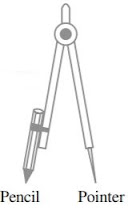Description: A pair – a pointer on one end and a pencil on the other.
Use: To mark off equal lengths but not to measure them. To draw arcs and circles.

• The DividerDescription: A pair of pointers
Use: To compare lengths.

• Set SquaresDescription: Two triangular pieces – one of them has 45°, 45°, 90° angles at the vertices and the other has 30°, 60°, 90° angles at the vertices.
Use: To draw perpendicular and parallel lines.

• The ProtractorDescription: A semi-circular device graduated into 180 degree-parts. The measure starts from 0° on the right hand side and ends with 180° on the left hand side and vice-versa.
Use: To draw and measure angles.

Circle

• Circle is closed plane figure consisting of all the points which are at a constant distance from a fixed point. This fixed point is called the centre and the distance of fixed point from the points on the circle is called radius of circle.

•  A line segment joining the two points of a circle is called chord of the circle.

• The chord passing through the centre is called diameter of a circle.

Construction of a circle when its radius is known

• Draw a circle of radius 3 cm.

Step I : Open the compass for the required radius of 3 cm.
Step II : Mark a point with a sharp pencil.
Step III : Place the pointer of the compass on O.
Step IV : Turn the compass slowly to draw the circle. Be careful to complete the movement amount in one instant.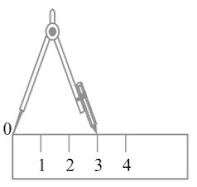Line Segment

• Line segment is a part of line that is bounded by two distinct end points and contains every point on the line between its end points.

Construction of a line segment of a given length using ruler and compass

Step I : Draw a line l. Mark a point A on a line l.
Step II : Place the compass pointer on the zero mark of the ruler. Open it to place the pencil point upto the 4.7 cm mark.
Step III : Taking caution that the opening of the compasses has not changed, place the pointer on A and swing an arc to cut l at B.
Step IV :is a line segment of required length.Constructing a copy of a given line segment using ruler and compass

Step I : Given, a line segmentwhose length is not known.
Step II : Fix the compass pointer on A and the pencil end on B. The opening of the instrument now gives the length of line segment.
Step III : Draw any line l. Choose a point C on l. Without changing the compasses setting, place the pointer on C.
Step IV : Swing an arc that cuts l at a point, say D. Now, line segmentis a copy of line segment.Perpendicular

• The property of being perpendicular (perpendicularity) is the relationship between how lines which meet at right angle (90 degrees). The property extends to other related objects. A line is said to be perpendicular to another line if the two lines intersect at right angle.

Drawing perpendicular to a line through a point on it, using ruler and a set-square

Step I : A line l and a point P are given as shown alongside.
Step III : Place a set-square with one of its edges along the already aligned edge of the ruler such that the right angled corner is in contact with the ruler.
Step IV : Slide the set-square along the edge of ruler until its right angled corner coincides with P.
Step V : Hold the set-square firmly in this position. Drawalong the edge of the set-square, where PQ is perpendicular to l.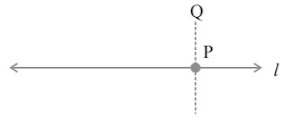Drawing perpendicular to a line through a point on it, using ruler and compass.

Step I : Let a point P on a line l.
Step II : With P as centre and a convenient radius, construct an arc intersecting the line l at two points A and B.
Step III : With A and B as centres and a radius greater than AP construct two arcs, which cut each other at Q.
Step VI : Join. Then, is perpendicular to I. We write⊥ l.Drawing a perpendicular to a line through a point not on it, using ruler and set-square.

Step I : Let l be the given line and P be a point outside l.
Step II : Place a set-square on l such that one arm of its right angle aligns along l.
Step III : Place a ruler along the edge opposite to the right angle of the set-square.
Step IV : Hold the ruler fixed. Slide the set-square along the ruler till the point P touches the other arm of the set-square.
Step V : Join PM along the edge through P meeting l at M. Now, PM I.Drawing a perpendicular to a line through a point not on it, using ruler and compass.

Step I : Given a line l and a point P not on it.
Step II : With P as centre, draw an arc, which intersects line l at two points A and B.
Step III : Using the same radius and with A and B as centres, construct two arcs that intersect at a point, (say Q) on the other side.
Step IV : Join PQ. Thus, PQ is perpendicular to l.Perpendicular bisector of a line segment

• A perpendicular bisector of a line segment is a line segment perpendicular to line segment and passing through the mid point. The perpendicular bisector of a line segment can be constructed using a compass by drawing circles centred at and with radius and connecting their two intersections.

Constructing perpendicular bisector by using ruler and compasses

Step I : Draw a line segmentof any length.
Step II : With A as centre, using compasses, draw a circle. The radius of your circle should be more than half the length of.
Step III : With the same radius and with B as centre, draw another circle using compasses. Let it cut the previous circle at C and D.
Step IV : Joinwhich cutsat O, use the divider to verify that O is the mid-point of. Also, Verify that ∠COA and ∠COB are right angles. Therefore, CD is the perpendicular bisector of.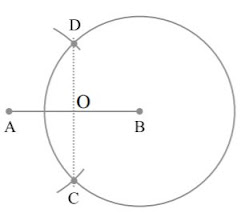Angle

• An angle is a figure formed by two rays, called the sides of the angle, sharing a common end
point, called the vertex of the angle.

Constructing an angle of a given measure

Make an angle of measure 40°.

Step I : Drawof any length.
Step II : Place the centre of the protractor at A and the zero edge along.
Step III : Start with zero near B. Mark point C at 40°.
Step IV : Join AC. ∠ BAC is the required angle.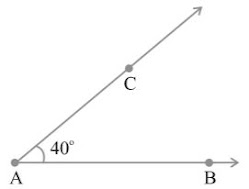Constructing a copy of an angle of unknown measure

Let ∠ A be an angle whose measure is not known.

Step I : Draw a line l and choose a point P on it.
Step II : Place the compasses at A and draw an arc to cut the rays of ∠ A at B and C.
Step III : Use the same compasses setting to draw an arc with P as centre, cutting l at Q.
Step IV : Set your compasses to the length BC with the same radius.
Step V : Place the compasses pointer at Q and draw the arc, to cut the arc drawn earlier in R.
Step VI : Join PR. This gives us ∠ P. It has the same measure as ∠ A.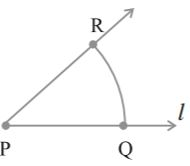Bisector of an angle

• The bisector of an angle is a ray whose end point is a vertex of the angle and which divides the angle into two equal angles.

Construction of bisector of an angle using ruler and compasses

Step I : With A as centre and using compasses, draw an arc that cuts both rays of ∠ A. Label the points of intersection as B and C.
Step II : With B as centre, draw (in the interior angle of ∠ A) an arc, whose radius is more than half the length of BC.
Step III : With the same radius and with C as centre, draw another arc in the interior angle of ∠ A.Angles of special measures

• There are some elegant and accurate methods to construct some angles of special measures, which do not require the use of the protractor.